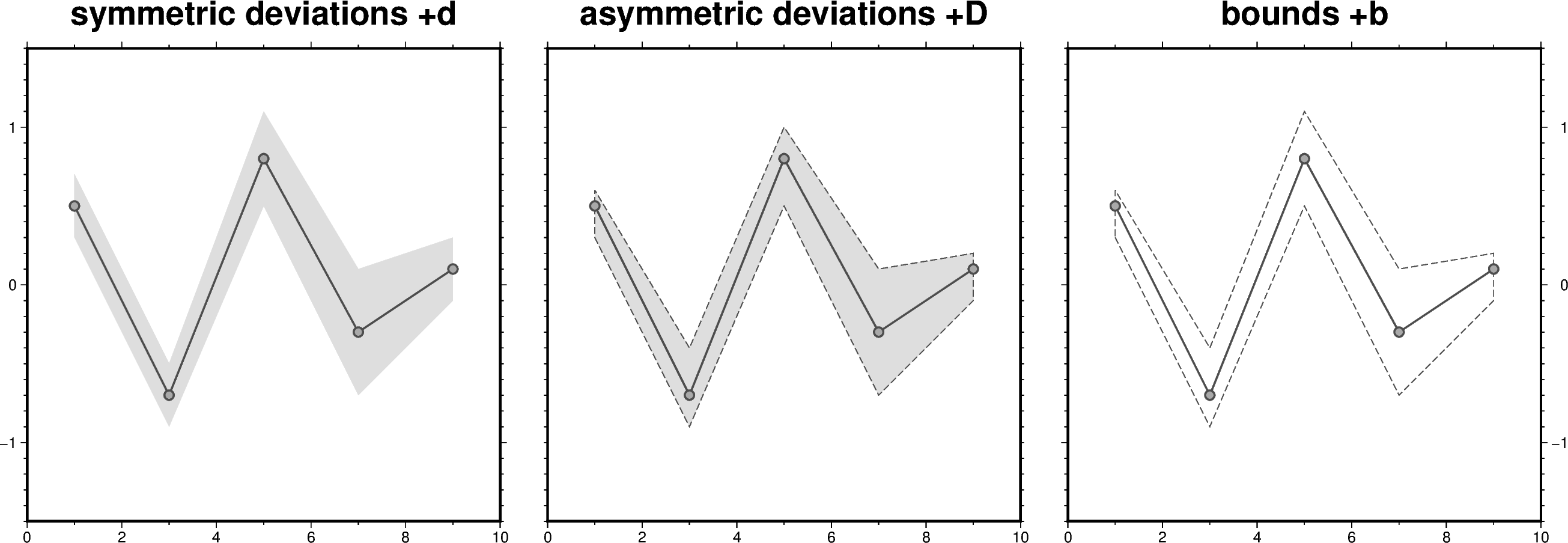# Envelope

The `close` parameter of the `pygmt.Figure.plot` method can be used to build a symmetrical or an asymmetrical envelope. The user can give either the deviations or the bounds in y-direction. For the first case append `"+d"` or `"+D"` and for the latter case `"+b"`.```import pandas as pd
import pygmt

# Define a pandas DataFrame with columns for x and y as well as the
# lower and upper deviations
df_devi = pd.DataFrame(
data={
"x": [1, 3, 5, 7, 9],
"y": [0.5, -0.7, 0.8, -0.3, 0.1],
"y_deviation_low": [0.2, 0.2, 0.3, 0.4, 0.2],
"y_deviation_upp": [0.1, 0.3, 0.2, 0.4, 0.1],
}
)

# Define the same pandas DataFrame but with lower and upper bounds
df_bound = pd.DataFrame(
data={
"x": [1, 3, 5, 7, 9],
"y": [0.5, -0.7, 0.8, -0.3, 0.1],
"y_bound_low": [0.3, -0.9, 0.5, -0.7, -0.1],
"y_bound_upp": [0.6, -0.4, 1.1, 0.1, 0.2],
}
)

# Create Figure instance
fig = pygmt.Figure()

# -----------------------------------------------------------------------------
# Left
fig.basemap(
region=[0, 10, -1.5, 1.5],
projection="X10c",
frame=["WSne+tsymmetric deviations +d", "xa2f1", "ya1f0.1"],
)

# Plot a symmetrical envelope based on the deviations ("+d")
fig.plot(
data=df_devi,
close="+d",
# Fill the envelope in gray color with a transparency of 50 %
fill="gray@50",
pen="1p,gray30",
)

# Plot the data points on top
fig.plot(
data=df_devi,
style="c0.2c",  # Use circles with a diameter of 0.2 centimeters
pen="1p,gray30",
fill="darkgray",
)

# Shift plot origin 11 centimeters in x direction
fig.shift_origin(xshift="11c")

# -----------------------------------------------------------------------------
# Middle
fig.basemap(
region=[0, 10, -1.5, 1.5],
projection="X10c",
frame=["WSne+tasymmetric deviations +D", "xa2f1", "yf0.1"],
)

# Plot an asymmetrical envelope based on the deviations ("+D")
fig.plot(
data=df_devi,
fill="gray@50",
# Add an outline around the envelope
# Here, a dashed pen ("+p") with 0.5-points thickness and
# "gray30" color is used
close="+D+p0.5p,gray30,dashed",
pen="1p,gray30",
)

# Plot the data points on top
fig.plot(data=df_devi, style="c0.2c", pen="1p,gray30", fill="darkgray")

# Shift plot origin 11 centimeters in x-direction
fig.shift_origin(xshift="11c")

# -----------------------------------------------------------------------------
# Right
fig.basemap(
region=[0, 10, -1.5, 1.5],
projection="X10c",
# Use "\\053" to handle "+b" as a string not as a modifier
frame=["wSnE+tbounds \\053b", "xa2f1", "ya1f0.1"],
)

# Plot an envelope based on the bounds ("+b")
fig.plot(data=df_bound, close="+b+p0.5p,gray30,dashed", pen="1p,gray30")

# Plot the data points on top
fig.plot(data=df_bound, style="c0.2c", pen="1p,gray30", fill="darkgray")

fig.show()
```

Total running time of the script: ( 0 minutes 1.588 seconds)

Gallery generated by Sphinx-Gallery